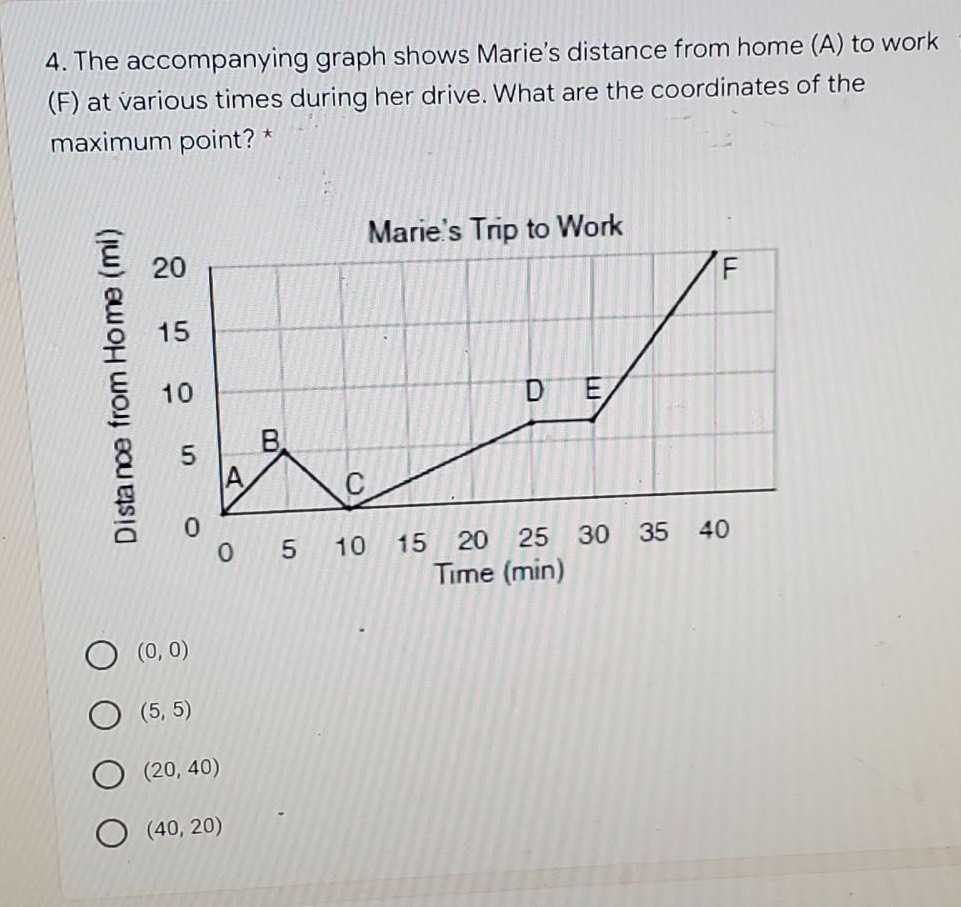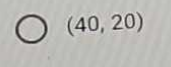### ¿Todavía tienes preguntas de matemáticas?

Pregunte a nuestros tutores expertos
Algebra
Pregunta4. The accompanying graph shows Marie's distance from home $$( A )$$ to work (F) at various times during her drive. What are the coordinates of the maximum point? *

$$( 0,0 )$$

$$( 5,5 )$$

$$( 20,40 )$$

$$( 40,20 )$$